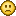### Grid filling line drawing thingamajig

#### letmethink

Hey all,

I'm not sure how use useful this will be for anyone, but I thought I might share since I found making this fun. Basically, you input an array, and this function will return one option for the lines required to cover this full array in the format of [rows, columns]. I have used it for a programmatic implementation of the Hungarian algorithm, and I thought I should share this part of it.

Below is an interactive demonstration of it working. (click to generate a new grid).

<a href="http://static.stencyl.com/games/33834-0.swf" target="_blank" class="new_win">http://static.stencyl.com/games/33834-0.swf</a>

Code: [Select]
`function clone(source:Dynamic){     var myBA:ByteArray = new ByteArray();     myBA.writeObject(source);     myBA.position = 0;     return(myBA.readObject()); }public function getSolution(grid:Array<Array<Int>>): Array<Array<Int>>{ grid = clone(grid); var count = 0; var count2 = 0; var valid = false; var found = false; var columns:Array<Int> = new Array(); var myValid = true; var myValid2 = true; for (e in 0...grid.length) columns.push(0); var rows:Array<Int> = new Array(); for (e in 0...grid.length) rows.push(0); count2 = 0; for (row in grid) { count = 0; for (item in row) { if (item == 0) { found = true; myValid = true; var count3 = 0; for (row2 in grid) { if (row2[count] == 0 && count3 != count2) { myValid = false; break; } count3 += 1; } var count3 = 0; myValid2 = true; for (item2 in grid[count2]) { if (item2 == 0 && count3 != count) { myValid2 = false; break; } count3 += 1; } if (myValid || myValid2) { valid = true; break; } } if (valid) break; count += 1; } if (valid) break; count2 += 1; } while (found) { if (!valid) { myValid = true; myValid2 = true; count2 = 0; for (row in grid) { count = 0; for (item in row) { if (item == 0) { valid = true; } if (valid) break; count += 1; } if (valid) break; count2 += 1; } } else { if (myValid && myValid2) myValid2 = false; } if (myValid) { rows[count2] = 1; count = 0; for (item in grid[count2]) { if (item == 0) { grid[count2][count] = -1; } count += 1; } } if (myValid2) { columns[count] = 1; count2 = 0; for (row in grid) { if (row[count] == 0) { grid[count2][count] = -1; } count2 += 1; } } found = false; valid = false; count2 = 0; for (row in grid) { count = 0; for (item in row) { if (item == 0) { found = true; myValid = true; var count3 = 0; for (row2 in grid) { if (row2[count] == 0 && count3 != count2) { myValid = false; break; } count3 += 1; } var count3 = 0; myValid2 = true; for (item2 in grid[count2]) { if (item2 == 0 && count3 != count) { myValid2 = false; break; } count3 += 1; } if (myValid || myValid2) { valid = true; break; } } if (valid) break; count += 1; } if (valid) break; count2 += 1; } trace(grid); } return [rows, columns];}`
~Letmethink

#### SadiQ

That is a really long function. What exactly is it trying to solve? It sounds interesting.
Proud member of the League of Idiotic Stencylers! Doing things in Stencyl that probably shouldn't be done.

#### letmethink

It can be used to determine whether you have obtained the optimal solution when solving an assignment problem using the Hungarian algorithm

It looks like it doesn't quite work some of the time so I will look into this.

« Last Edit: March 20, 2016, 03:08:21 am by letmethink »
~Letmethink

#### SadiQ

I read the wikipedia article before I posted (and I didn't understand much passed the first couple of lines), yet I wasn't able to figure out what exactly you want to achieve in that test game you posted.
So far it looks like you're just placing some circles in the grid, but I don't understand the rules of the placement.
Proud member of the League of Idiotic Stencylers! Doing things in Stencyl that probably shouldn't be done.

#### letmethink

The circles are placed randomly, but the function decides how to place lines to cover the grid in the optimal number of lines so that all 0s are covered.
~Letmethink

#### SadiQ

Interesting. I'll have to look into it when I have some time available. I wonder if it can be applied in games (AI), but from the complexity...it might not help muchProud member of the League of Idiotic Stencylers! Doing things in Stencyl that probably shouldn't be done.